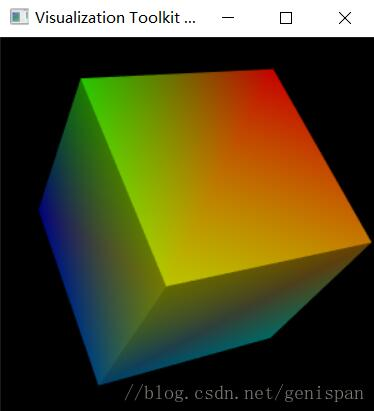python绘制立方体的方法

```#!/usr/bin/env python

# This is (almost) a direct C++ to Python transliteration of
# <VTK-root>/Examples/DataManipulation/Cxx/Cube.cxx from the VTK
# source distribution, which "shows how to manually create vtkPolyData"
#
# A convenience function, mkVtkIdList(), has been added and one if/else
# so the example also works in version 6 or later.
#
# Lines like `obj->Delete()` have been transliterated as `del obj` to,
# preserve the resemblance to the original C++ example, although I
# doubt this achieves anything beyond what Python's garbage collection
# would do anyway.

import vtk

# Makes a vtkIdList from a Python iterable. I'm kinda surprised that
# this is necessary, since I assumed that this kind of thing would
# have been built into the wrapper and happen transparently, but it
# seems not.

def mkVtkIdList(it):
vil = vtk.vtkIdList()
for i in it:
vil.InsertNextId(int(i))
return vil

# 绘制通用方法
def myShow(cube):
# Now we'll look at it.
cubeMapper = vtk.vtkPolyDataMapper()
if vtk.VTK_MAJOR_VERSION <= 5:
cubeMapper.SetInput(cube)
else:
cubeMapper.SetInputData(cube)
cubeMapper.SetScalarRange(0, 7)
cubeActor = vtk.vtkActor()
cubeActor.SetMapper(cubeMapper)

# The usual rendering stuff.
camera = vtk.vtkCamera()
camera.SetPosition(1, 1, 1)
camera.SetFocalPoint(0, 0, 0)

renderer = vtk.vtkRenderer()
renWin = vtk.vtkRenderWindow()

iren = vtk.vtkRenderWindowInteractor()
iren.SetRenderWindow(renWin)

renderer.SetActiveCamera(camera)
renderer.ResetCamera()
renderer.SetBackground(0, 0, 0)

renWin.SetSize(300, 300)

# interact with data
renWin.Render()
iren.Start()
del cubeMapper
del cubeActor
del camera
del renderer
del renWin
del iren

def main():
# x = array of 8 3-tuples of float representing the vertices of a cube:
# 8个三维值代表长方体的8个顶点
x = [(0.0, 0.0, 0.0), (1.0, 0.0, 0.0), (1.0, 1.0, 0.0), (0.0, 1.0, 0.0),
(0.0, 0.0, 1.0), (1.0, 0.0, 1.0), (1.0, 1.0, 1.0), (0.0, 1.0, 1.0)]

# pts = array of 6 4-tuples of vtkIdType (int) representing the faces
#  of the cube in terms of the above vertices
# 点的编号0-7，每个面由4个点组成
pts = [(0, 1, 2, 3), (4, 5, 6, 7), (0, 1, 5, 4),
(1, 2, 6, 5), (2, 3, 7, 6), (3, 0, 4, 7)]

# We'll create the building blocks of polydata including data attributes.
cube = vtk.vtkPolyData()
points = vtk.vtkPoints()
polys = vtk.vtkCellArray()
scalars = vtk.vtkFloatArray()

# Load the point, cell, and data attributes.
for i in range(8):
points.InsertPoint(i, x[i])
for i in range(6):
polys.InsertNextCell(mkVtkIdList(pts[i]))
for i in range(8):
scalars.InsertTuple1(i, i)

# We now assign the pieces to the vtkPolyData.
cube.SetPoints(points)
del points
cube.SetPolys(polys)
del polys
cube.GetPointData().SetScalars(scalars)
del scalars

myShow(cube)
# Clean up
del cube

main()```